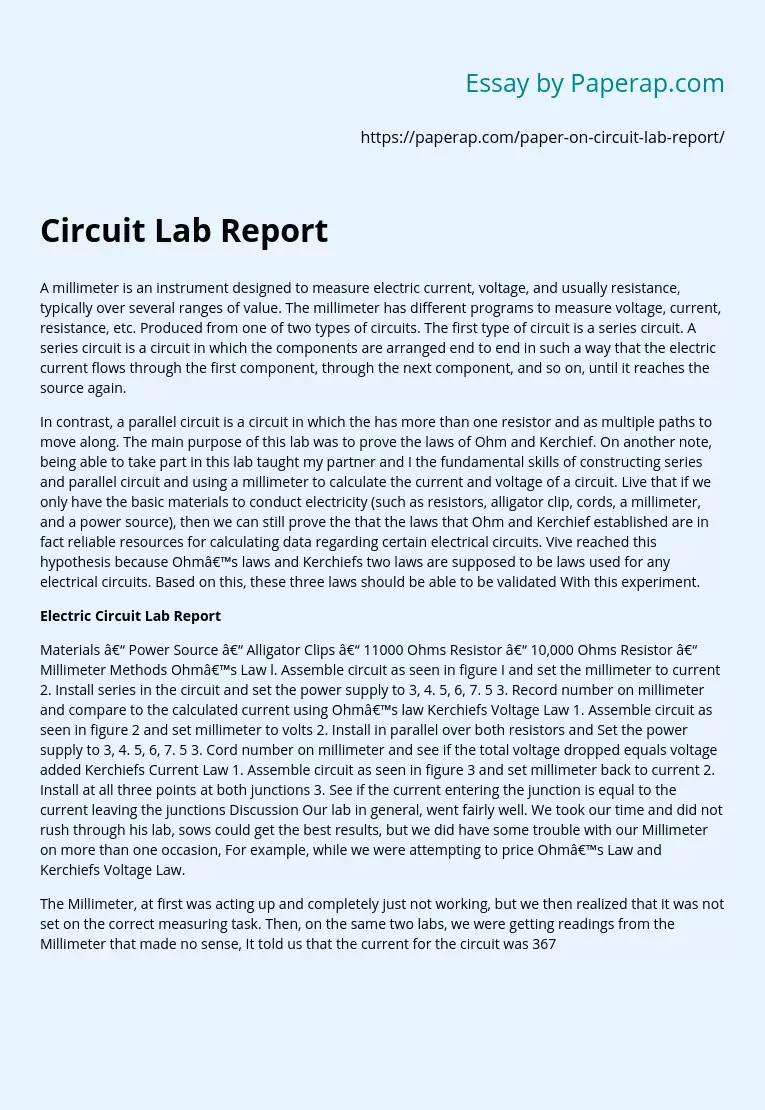# Electric Circuit Lab Report

A millimeter is an instrument designed to measure electric current, voltage, and usually resistance, typically over several ranges of value. The millimeter has different programs to measure voltage, current, resistance, etc. Produced from one of two types of circuits. The first type of circuit is a series circuit. A series circuit is a circuit in which the components are arranged end to end in such a way that the electric current flows through the first component, through the next component, and so on, until it reaches the source again.

In contrast, a parallel circuit is a circuit in which the has more than one resistor and as multiple paths to move along. The main purpose of this lab was to prove the laws of Ohm and Kerchief. On another note, being able to take part in this lab taught my partner and I the fundamental skills of constructing series and parallel circuit and using a millimeter to calculate the current and voltage of a circuit.

Live that if we only have the basic materials to conduct electricity (such as resistors, alligator clip, cords, a millimeter, and a power source), then we can still prove the that the laws that Ohm and Kerchief established are in fact reliable resources for calculating data regarding certain electrical circuits. Vive reached this hypothesis because Ohm’s laws and Kerchiefs two laws are supposed to be laws used for any electrical circuits. Based on this, these three laws should be able to be validated With this experiment.

Get quality help nowProf. FinchVerified

Proficient in: Electrical Engineering4.7 (346)

“ This writer never make an mistake for me always deliver long before due date. Am telling you man this writer is absolutely the best. ”+84 relevant experts are online

## Electric Circuit Lab Report

Materials – Power Source – Alligator Clips – 11000 Ohms Resistor – 10,000 Ohms Resistor – Millimeter Methods Ohm’s Law l. Assemble circuit as seen in figure I and set the millimeter to current 2. Install series in the circuit and set the power supply to 3, 4. 5, 6, 7. 5 3. Record number on millimeter and compare to the calculated current using Ohm’s law Kerchiefs Voltage Law 1. Assemble circuit as seen in figure 2 and set millimeter to volts 2. Install in parallel over both resistors and Set the power supply to 3, 4. 5, 6, 7. 5 3. Cord number on millimeter and see if the total voltage dropped equals voltage added Kerchiefs Current Law 1. Assemble circuit as seen in figure 3 and set millimeter back to current 2. Install at all three points at both junctions 3. See if the current entering the junction is equal to the current leaving the junctions Discussion Our lab in general, went fairly well. We took our time and did not rush through his lab, sows could get the best results, but we did have some trouble with our Millimeter on more than one occasion, For example, while we were attempting to price Ohm’s Law and Kerchiefs Voltage Law.

The Millimeter, at first was acting up and completely just not working, but we then realized that it was not set on the correct measuring task. Then, on the same two labs, we were getting readings from the Millimeter that made no sense, It told us that the current for the circuit was 367. 83 Ohms which for the type of circuit that we built, was impossible. After fixing the problem with the Millimeter and proving Ohm’s Law and Kerchiefs Voltage Law, eve moved onto the Current Law that Kerchief established. This time, the problem wasn’t the Millimeter, or the resistors, or the power supply, or anything else.

It avgas us. We were overall a bit confused on how the circuit was created and it took a long time to eventually construct and then prove. Even though the problems were an annoyance, the lab was very insightful and taught us a lot about electric currents and circuits. Conclusion At the conclusion of this experiment, our results supported our hypothesis greatly even though our numbers were not a hundred percent on point. But here is room for error, like the fact that the power supply was not great quality and doesn’t give exactly 3 volts or 4. Volts or any of that, Also, the resistors are not high-quality resistors and are also worn down trot years of use, so they don’t give exactly 11000 / 10,000 Ohms like we were looking for. Other sources tot error included the Millimeter, which was not exact because the Millimeter rounds numbers, the Alligator clips, which are (like the power supply and resistors) not the best and shed energy, and our calculations, which could have suffered from any addition or multiplication or division error,